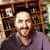# Calculate sum of value in different repeats

160
3
12-06-2021 08:58 AMby
New Contributor III

Hi, I don't understand this. I think I've been trying everything at this point, but I guess I did not.

I have multiple repeats, which all creates a value for Example costs. That could be in a grocery store, where we can choose fruits and fish. Under that we have a level with types, that could be bananas, apples, salmon etc.

All have a value of the weight. I want to calculate the total  weight of all the groceries. I can get this to work with connect, but not in web.

This seems to work in connect:

coalesce(sum(\${weight_1}), 0) + coalesce(sum(\${weight_2}), 0)+ coalesce(sum(\${weight_3}), 0)

But the field is blanc in web.

I then tried to add the raw calculations in 3 fields like:
sum(\${weight_1})

sum(\${weight_2})

sum(\${weight_3})

And added to a more simple version:
sum(\${weight_1})+sum(\${weight_2})+sum(\${weight_3})

That actually seemed to work, when I did the first testing also in web, but when I hided and added more calculations to it, or maybe it was since I used multiple calcualtions in the same category, it again didn't calculated the sum.

Any idea how to create this?
Thanks

3 RepliesbyEsri Notable Contributor

Hi @kelin84,

We have made some updates in the 3.14 beta builds of Connect/field which will support calculationModes and better calculations and expressions when using repeats and the indexed-repeat function. This may help with you case.

These new enhancements and fixes can be tested in the latest 3.14 beta builds available on EAC later today.

Regards,

Phil.by
New Contributor III

Hi, thank you Philip, that will for sure make it more intuitive in the future. I'm not going to test it now, since I found a working solution. Which I'll write below 🙂by
New Contributor III

Most often, you I just need to check the samples.

Now I can't find which one it was, but with the use of Javascript&colon;

function smartsum(...values) {

var total = 0;

for (var value of values) {

if (isFinite(value)) {

total += Number(value);

}

}

}

And then calculation in the Spreadsheet will work:

pulldata("@javascript", "functions.js", "smartsum", \${number1}, \${number2})

This one can jut be extended...